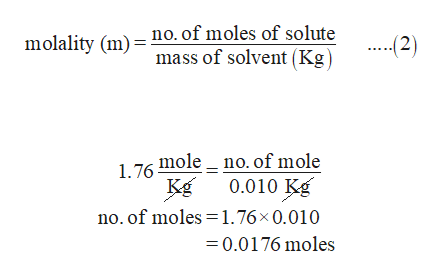# A solution containing 3.30 g  of an unknown nonelectrolyte liquid and 10.00 g  water has a freezing point of −3.33 °C. The Kf = 1.86 °C/m for water. Calculate the molar mass of the unknown liquid in g/mol.Answer:_____ g/mol

Question
3 views

A solution containing 3.30 g  of an unknown nonelectrolyte liquid and 10.00 g  water has a freezing point of −3.33 °C. The Kf = 1.86 °C/m for water. Calculate the molar mass of the unknown liquid in g/mol.

check_circle

Step 1

When the non-electrolytes liquid is added in solution there is the change in freezing point which is given 3.33°C and freezing point constant Kf is 1.86 °C/m for water.

Now for finding the molecular mass of unknown non-electrolyte liquid, we have to first find molality of unknown non-electrolyte liquid by using equation 1

Substitutes 3.33°C for ΔTf; 1.86 °C/m for Kf  in equation 1

Step 2

Since molality is the measure of no.of moles per kg of solvent

So m = 1.76 mole per kg

Mass of solvent is given 10 g, So converts it into kilogram

1kg =1000 g

1 g = 10-3 Kg

10...help_outlineImage Transcriptionclosemolality (m) no. of moles of solute mass of solvent (Kg ...-2) no. of mole 0.010 Kg 1 76 mole no. of moles 1.76x 0.010 =0.0176 moles fullscreen

### Want to see the full answer?

See Solution

#### Want to see this answer and more?

Solutions are written by subject experts who are available 24/7. Questions are typically answered within 1 hour.*

See Solution
*Response times may vary by subject and question.
Tagged in

### Solutions